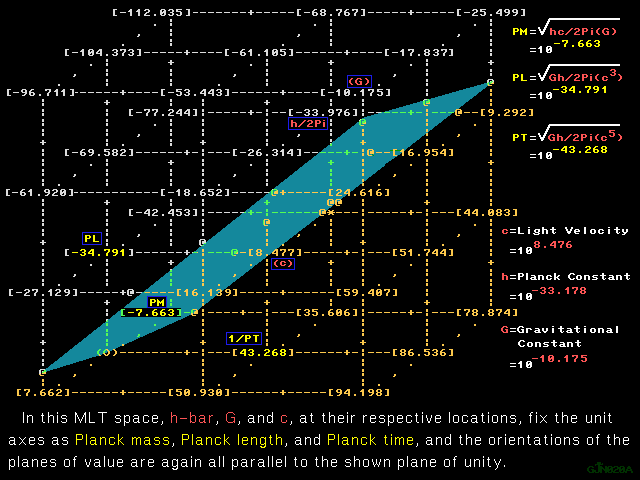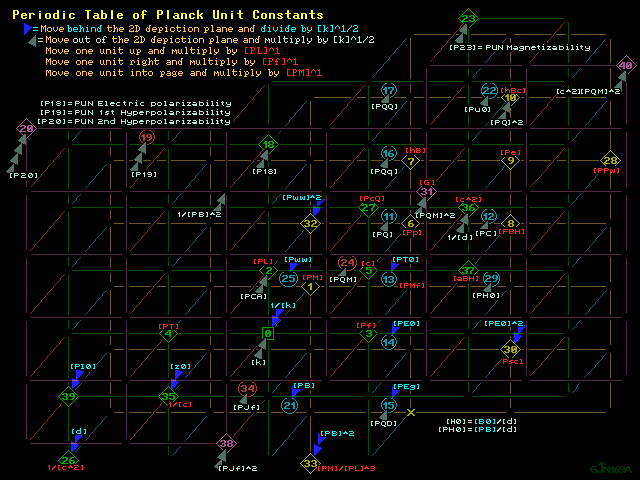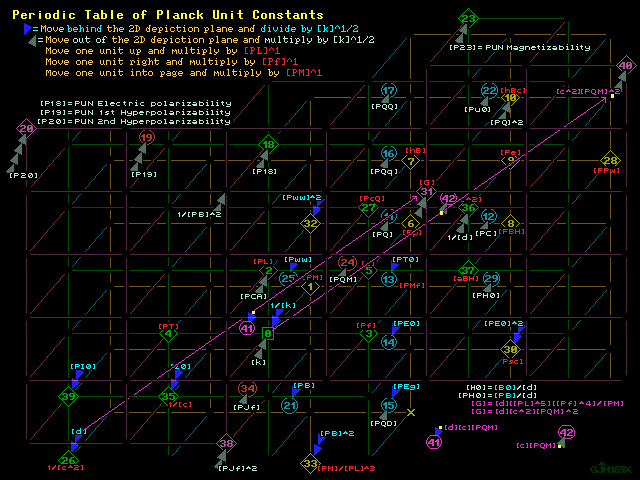```Color print   Values table   1280 X 960 GIF   Grayscale print

Tutorial back, Atomic constants map    Symbol key    Tutorial end

Wedges as displacement into the fourth dimensionColor print      1280 X 960 GIF      B & W printColor print      1280 X 960 GIF      B & W print

The Periodic Table of Planck Unit Constants
Determines the Exact Value of the Newtonian Gravitational Constant

Numerical patterns used to extrapolate unseen marks on slide rule
scales and unlisted values in logarithm tables for hundreds of years
combine in this periodic table of fundamental constants to show that
only one numerical value for the Newtonian gravitational constant
aligns the pervasive patterns of near misses among the constants
into exact numerical matches.  The mismatches found in the quantity
calculus archive for any other [G] value reaffirm that proof:

the exact numerical value of the Newtonian gravitational constant is

6.6917625607...e-11 = 10^-10.17445947721306... = T-10.1744595

Numbers between dollar signs below refer to locations in graphics
above : projections of that vector space structuring into a lattice
of logic the values of the Planck unit constants with the magnitudes
expressed as common logarithms in 2D portrayals of a 3D map, but
including representation of displacement into 4D.  Multiplication by
the factor {[k]^[1/2]} is signified by a wedge expanding in front
of the 2D depiction plane, toward the viewer, and division by that
factor of {[k]^[1/2]} is represented by a wedge expanding away from
view, toward being behind the 2D depiction plane:

Moving two wedges toward in front of the image is multiplication by
electric constant [k] (= permittivity) in (Farad per Meter)^+1 and
moving two wedges toward being behind the image is division by [k]

It is important to note that in the context of the atomic units
and their mappings, GDA discussion alludes to multiplication by the
Coulomb constant [Ke] = 1/{[4Pi][k]} represented by displacement in
4D as if moving behind the depiction plane while discussion in the
context of these Planck unit spaces alludes to multiplication by [k]
represented by displacement in the opposite direction, toward being
in front of the depiction plane.  This is just different terminology
for the same conceptual situation with one important advantage:
because the square of charge is defined in the atomic context as
[action][velocity]/[Coulomb constant] : {[Q0]^2} = [hB][v0]/[Ke]
with [Ke] = 1/{[4Pi][k]} and [v0] = [c][aa] , much of that vector
space is populated by constants with tweaks : occurrences of one
constant which has units combined with one or more constants which
have no units.

Defining [Planck charge]^2 as [action][velocity][k] = [hB][c][k] :

{[PQ]^2} = [hB][c][k] and not {[PQ]^2} = [hB][c]/({[4Pi][k]}^-1) ,

the Planck unit vector space is rendered both free of tweaks and
free of the need to discuss and consider reciprocals of reciprocals.
Since customary, popular Planck charge [PX] is defined by

[PX]^2 = [hB][c]/[Ke] , this means that {[PQ]^2} = {[PX]^2}/[4Pi] .

This choice is important in the maps because it bestows a singular
elegance upon the definition of that group of relationships, that
class of vectors wherein lies the quintessence of determining the
one value that the Newtonian gravitational constant [G] must have.

In the triplet of graphics immediately above, GJN169X, GJN169Y,
and GJN169Z, the Plank constants vector space is reproduced with an
emphasis on that vector which proceeds from the plot point for the
magnetic constant [d] (= permeability) to [G], the same vector and
relationship represented by displacement from the origin to two
wedges in front of location \$40\$ in the map.  That vector's identity

is [k]{[PL]^5}{[Pf]^4}/[PM] , it is [k][c^4][PL]/[PM] , and that

vector's identity is

{[PQ][PL]}^2
[c^2]{[PQ]^2}/{[PM]^2} =  --------      :
{[PM][PT]}^2

by {[PM}^2} , square of the Planck mass value that will be set by
adjustment of the value of [G] .

With the Planck constants space modeled on the template of the self
validating atomic constants space, where pre-existing, pre-defined,
unadjustable constants populate, singly or in combinations, a space
which is coherent, the question here is whether [G] is to have that
one numerical value that will endow the Planck space with that same
state of coherency.  Either one accepts that the highlighted vector
and all those identical to it have exactly the same numerical value
as the square of the fine structure constant and that this space is
coherent, or this Planck constants space consists partly of vectors
with the numerical values of constants and partly of vectors with
values which are only near misses to those of familiar constants.

History records that the development and acceptance of the periodic
table of the elements in chemistry included the completion of a
pattern, a missing element that was later observed in the spectrum
of the sun and thus named helium when attained.  The completion of
pattern here, too, is too elegant to be wrong.  Due to the precedent
of coherence in the atomic units vector space, due to the pervasive
numerical coincidences found in both these graphics and in the
quantities archive, and especially due to the identities of many of
the coincidences involved, it is unreasonable to accept any other
value for [G] .  The vector emphasized in the maps above must have
the numerical value of the square of the fine structure constant at
10^-4.2736694 and [G] must have the numerical value 10^-10.1744595 :

{[PQ][PL]}^2
--------    <=> {[aa]^2}  ,  and  [G] <=> [d]{[aa]^2}  .
{[PM][PT]}^2

In judging the evidence for a [G] value that differs so considerably
from most laboratory experiment results, it is important to remember
the scope of the problem.  A typical textbook on physics discusses
a mass of 215 kg on the surface of the earth endowed with a charge
identical in magnitude to an opposing charge at earth's center so
that the gravitational attraction by the entire mass of the planet
is offset.  In his book Six Easy Pieces, Richard Feynman explains
how two grains of sand thirty meters apart would attract each other
with an electrical force of three million tons without a balancing
of charges, if instead of likes repelling everything attracted
everything else.  Still another thought experiment might make the
point more succinctly.

As stated by that same Nobel prize winner in that same book, the
ratio of gravitational attraction relative to the electrical
repulsion between two electrons is 1/[4.17 x 10^42] : 10^-42.6+...
In a standard static electricity setup involving two small masses
with equal and like charges hung by insulating threads, the force
[F] of repulsion is calculated to be the Coulomb constant [Ke]
multiplied by the square of the charges [Q]^2, all divided by the
square of the distance [L] between them: [F] = [Ke]{[Q]^2}/{[L]^2} .
If the initial distance is one centimeter and that force is to be
reduced to the strength of the gravitational force between those two
masses by altering only [L] ,

[L] cannot be made one meter :

1/{[10^2] x [10^2]} => [F] x [10^-4] ,

[L] cannot be made one kilometer :

1/{[10^5] x [10^5]} => [F] x [10^-10] ,

[L] cannot be made one hundred million kilometers
= 2/3 of the distance to the sun = .66 astronomical units :

1/{[10^13] x [10^13]} => [F] x [10^-26] ,

[L] cannot be made one hundred million times as far as .66 A U
= 66 million astronomical units :

1/{[10^21] x [10^21]} => [F] x [10^-42] ,

[L] must be at least twice that far,
[L] must be at least two hundred million times as far as .66 A U:

1/{[10^21.3] x [10^21.3]} => [F] x [10^-42.6] .

If that commonly exhibited, hands on, observational experiment of
the electrostatic force is to be altered for observation of a force
comparable to that of the gravitational force by changing only their
distance of separation, those two little masses on threads must be
more than 132 million astronomical units apart.

While such an experiment is unlikely to take place, if it did, at
least the experimenters would not be using relatively minute masses
to emulate celestially observed gravitational interactions while
immersed deep in the gravity well of one of those celestial bodies,
trying to obtain as good a vacuum as possible, estimating outgassing
properties of materials, and/or torsion factors of fibers, and/or
temperature fluctuations, and/or tidal influences while they stand
in a pair of painted footprints.

Finally, there is one more thing to keep in mind.  With every new
publication of CODATA values, it must be said again: there exists no
recognized relationship between the Newtonian gravitational constant
and any of the other constants.  All the constants exist in the same
universe and therefore have some kind(s) of relationship(s), but
experiments involving forces 10^42 times as weak as tabletop setups
will be conducted as if such relationships did not exist and cannot
be affecting results.

Using one dimension or more, do the math.  Decide for yourself.

GUNTERTABLE3 only print
---------------------------------++---------------------------------
GUNTERTABLE3 : for the Periodic Table of Planck Unit Constants
---------------------------------++---------------------------------
POWER OF TEN | COMMENTS, NOTES                                 UNITS
---------------------------------++---------------------------------
AUN = Atomic unit of                          PUN = Planck unit of

0.0000000  1 = ^0 = [Anything]^0
\$0\$  = {^0}{[PM]^0}{[PL]^0}{[Pf]^0}{{[k]^0}               ORIGIN

- 11.0528513  [k] = 1/{[4Pi][Ke]}          Fradmetr=Cuulhrtzmetrwebr
= [Q0]/{4[w0][v0]} = {[Jf]^2}[Me][a0][Pi]/4        hnryh2tzmetr
= {[aa]^2}/([d]{[v0]^2})    = [10^7][Meter]/([4Pi][c^2][Henry])
= {[aa]^2}{[a0]^-2}{[2af]^-2}/[d]    = [AUN permittivity]/[4Pi]
= 1/{[c^2][d]} = 1/{2[v0][RK]} = 1/{2[aa][c][RK]}  hrtzmetrohmm
\$0\$  = [k] = ({[PL][Pf]}^-2)/[d]  = Electric constant = permittivity

-  7.6628219  [PM] = [PE]/[c^2] = {[Q0]^2}[d]/{[4Pi][aa][PL]}   Kgrm
= [Me][a0][aa]/[PL] = {[aa]^2}[Me][Pf]/[2af] = [hB]/{[PL][c]}
= [z0]{[PQ]^2}/{[PL][c]} = [Pk][Bk][d][k] = [k]{[PL]^3}{[PB]^2}
= [z0]{[PC]^2}/({[PL]^2}{[Pf]^3}) = ({[Q0]^2}[Ke]/{[G][aa]})^.5
\$1\$  = [PM] = {[hB][c]/[G]}^.5                         = Planck Mass

- 34.7909227  [PL] = [G][PM]/[c^2] = [a0][Me][aa]/[PM]          Metr
= [hB]/{[PM][c]} = [u0][Me]/{[c][Q0][PM]} = 1/{[z0][Pf][k]}
\$2\$  = [PL] = {[hB][G]/[c^3]}^.5                     = Planck length

- 45.8437740  [PCA] = [PQ]/[PT0] = {[PQ]^2}/[PE]                Frad
\$2\$  = [PL][k] = [PL]/{[4Pi][Ke]}                  = PUN capacitance

+ 43.2677434  [Pf] = [c]/[PL] = [PM][2af]/({[aa]^2}[Me])        Hrtz
= [PB]{[G][k]}^.5 = [hB]/({[PL]^2}[PM]) = ([G][PM]/{[PL]^3})^.5
\$3\$  = [Pf] = {[hB][G]/[c^5]}^-.5        = 1/[PT] = Planck frequency

- 43.2677434  [PT] = 1/[Pf] = {[aa]^2}[Me]/{[PM][2af]}     hrtz=Scnd
= [PL]/[c] = 1/([PB]{[G][k]}^.5) = ([G][PM]/{[PL]^3})^-.5
\$4\$  = [PT] = {[hB][G]/[c^5]}^.5 = {[PL]^2}[PM]/[hB]   = Planck time

+  8.4768207  [c] = [z0]/[d] = 1/{[k][z0]} = [v0]/[aa]      HrtzMetr
= [hB]/{[PM][PL]} = (1/{[k][d]})^.5   metrtslaVltt=Cuulf12dk12m
= [f0]/{2[Rc]} = [E0]/{[aa][B0]}          = [AUN velocity]/[aa]
\$5\$  = [PL][Pf]               = Light speed in vacuum = PUN velocity

+  0.8139988  [Pp] = [hB]/[PL] = [PM][c] = [PE]/[c]     HrtzKgrmMetr
\$6\$  = [PM][PL][Pf]                                = Planck momentum

- 33.9769238  [hB] = [h]/[2Pi] = [PM][PL][c]   hrtzJuul=HrtzKgrmM2tr
= {[Q0]^2}/{[4Pi][k][v0]} = [B0][Q0]{[a0]^2}       CuulM2trTsla
= {[Q0]^2}[Ke]/[v0] = [Q0][w0]/[Pi] = [He]/{[4Pi][Rc][c]}
= {[Q0]^2}[RK]/[2Pi] = [u0][Me]/[Q0] = [G]{[PM]^2}/[c]
*7*  = {[Q0]^2}[v0][d]/([4Pi]{[aa]^2}) = {[PQ]^2}[z0]   = AUN action
\$7\$  = [PM]{[PL]^2}[Pf] = [Me]{[a0]^2}[2af]             = PUN action

+ 44.0817422  [FBH] = [FPX] = [FPC] = [4Pi][FPQ] = [c^4]/[G]    Nwtn
= {[PQ]^2}/({[PL]^2}[k]) = [d]{[PC^2]} = [hB][c]/{[PL]^2}
= {[PX]^2}/({[PL]^2}[4Pi][k]) = [PE]/[PL] = [PPw]/[c]
= {[PM]^2}[G]/{[PL]^2} = [c^2][PM]/[PL] = [c][PM][Pf]
= {[Q0]^2}[Ke]/({[PL]^2}[aa]) = [z0][k][c^3][PM]/[PL]
= {[a0]^2}[Fe]/({[PL]^2}[aa]) = {[Fe]^2}[Mp]/([Me][Fg]{[aa]^4})
\$8\$  = [PM][PL]{[Pf]^2}                               = Planck force

+  9.2908195  [PE] = [PM][c^2]  = [FBH][PL]            Juul=MetrNwtn
\$9\$  = [PM]{[PL]^2}{[Pf]^2}                          = Planck energy

- 25.5001032  [hBc] = [hB][c] = [PM][PL][c^2]  C2ulfradMetr=JuulMetr
= {[PQ]^2}[Ke][4Pi] = {[PQ]^2}/[k]    A2prHnryMetr=H2tzKgrmM3tr
= {[PX]^2}[Ke] = ({[Me][2af]/[B0]}^2)/{[aa][4Pi][k]}   M2trNwtn
\$10\$ = [PM]{[PL]^3}{[Pf]^2} = [PE][PL] = [FBH]{[PL]^2} = [G]{[PM]^2}

- 36.5529545  {[PQ]^2} = [hB][c][k] = [G][k]{[PM]^2}   FradJuul=C2ul
= [k][c^2][PM][PL] = {[Q0]^2}/{[4Pi][aa]}          AmprKgrmtsla
= [Me][a0][aa]/[d] = [PM][PC]/[PB]             A2prKgrmMetrnwtn
= {[PM]^2}{[Pf]^2}/{[PB]^2}           hnryKgrmM2tr=H2tzK2rmt2la
= {[PX]^2}/[4Pi]            = {[Popular Planck charge]^2}/[4Pi]
\$10\$ = [PM]{PL]^3}{[Pf]^2}[k] = [PL][PM]/[d] = [GDA Planck Charge]^2

- 18.2764773  [PQ] = ({[hB][c][k]}^.5) = [Q0]/({[4Pi][aa]}^.5)  Cuul
= [PC][PT] = [PM][Pf]/[PB]                Amprhrtz=HrtzKgrmtsla
= [PX]/{[4Pi]^.5}          = [Popular Planck charge]/{[4Pi]^.5}
\$11\$ = {[PM]^.5}{[PL]^1.5}[Pf]{[k]^.5}           = GDA Planck charge

+ 24.9912662  [PC] = [PQ][Pf] = ([k]{[c]^3}[PM][Pf])^.5     CuulHrtz
= ({[hB][c]/[d]}^.5)/[PL] = {[FBH]/[d]}^.5    Ampr=F12dHrtzJ12l
= [PM][Pf]{[G][k]}^.5} = [Pf]{[hB][c][k]}^.5   F12dH2tzK12mMetr
= [Q0][Pf]/({[4Pi][aa]}^.5)                        h12yM12rN12n
\$12\$ = {[PM]^.5}{[PL]^1.5}{[Pf]^2}{[k]^.5}             = PUN current

+ 27.5672968  [PT0] = [PE]/[PQ] = [PB][PcQ] = [PC][z0]          Vltt
= [hB][k]/[PQ] = [PQ]/{[PL][k]} = [PH0]/{[k][Pf]}      cuulJuul
\$13\$ = {[PM]^.5}{[PL]^1.5}[Pf]{[k]^-.5}     = PUN electric potential

+ 62.3582195  [PE0] = [FBH]/[PQ] =  [PE]/{[PQ][PL]}         metrVltt
= [z0][PB]/[d] = [z0][PH0] =   [PQ]/([k]{[PL]^2})      cuulNwtn
= [z0][PB][c^2][k] = [c][PB] = [PC]/{[k][c][PL]}   Cuulfradmetr
\$14\$ = {[PM]^.5}{[PL]^-.5}[Pf]{[k]^-.5}         = PUN electric field

+ 86.0962908  [PQD] = [PQ]/{[PL]^3} = [c][PB][k]/[PL]       Cuulm3tr
\$15\$ = {[PM]^.5}{[PL]^-1.5}[Pf]{[k]^.5}         = PUN charge density

+ 97.1491421  [PEg] = [PB][Pf] = [PQ]/([k]{[PL]^3})         m2trVltt
= [FBH]/{[PQ][PL]} = [z0][PB]/{[d][PL]}   cuulmetrNwtn=HrtzTsla
\$15\$ = {[PM]^.5}{[PL]^-1.5}[Pf]{[k]^-.5}=PUN electric field gradient

- 53.0674000  [PQq] = [PQ][PL] = [hB]/{[PB][PL]}            CuulMetr
\$16\$ = {[PM]^.5}{[PL]^2.5}[Pf]{[k]^.5}  = PUN electric dipole moment

- 87.8583227  [PQQ} = [PQ]{[PL]^2} = [hB]/[PB]              CuulM2tr

-115.4256195  [P18] = [G][PM][k]/{[Pf]^2}      Kgrmt2la=C2uljuulM2tr
= [PQ]/{[PB][Pf]} = {[PQ]^2}{[PL]^2}/[PE]          Cuulhrtztsla
\$18\$ = {[PL]^3}[k] = [PM]/{[PB]^2}     = PUN electric polarizability

-177.7838390  [P19] = {[PQ]^3}{[PL]^3}/{[PE]^2}         C3ulj2ulM3tr
= [PE]/{[PE0]^3} = [P18]/[PE0]    = PUN 1st hyperpolarizability
\$19\$ = {[PM]^-.5}{[PL]^3.5}{[Pf]^-1}{[k]^1.5}

-240.1420585  [P20] = [PE]/{[PE0]^4} = [PQq]/{[PE0]^3}  C4ulj3ulM4tr
= ({[PQ][PL]}^4)/{[PE]^3} = [P18]/{[PE0]^2}        JuulM3trv3tt
\$20\$ = {[PM^-1}{[PL]^4}{[Pf]^-2}{[k]^2} =PUN 2nd hyperpolarizability

+ 53.8813988  [PB] = {[PM]/([k]{[PL]^3})}^.5 = [PC][d]/[PL]     Tsla
\$21\$ = {[PM]^.5}{[PL]^-1.5}{[k]^-.5}     = PUN magnetic flux density

- 44.5905793  [Pu0] = [PC]{[PL]^2} =[PQ][PL][c] =[PE]/[PB]  AmprM2tr
= [hB][c]{[PL][k]/[PM]}^.5         = PUN magnetic dipole moment
\$22\$ = {[PM]^.5}{[PL]^3.5}{[Pf]^2}{[k]^.5}

- 98.4719780  [P23] = [hB][c][k]{[PL]^2}/[PM]           C2ulkgrmM2tr
= [PE]/{[PB]^2} =  {[PQ]^2}{[PL]^2}/[PM]      Juult2la=hnryM4tr
\$23\$ = {[PL]^5}{[Pf]^2}[k]    = {[PL]^3}/[d]   = PUN magnetizability

- 10.6136554  [PQM] = [PQ]/[PM] = {[G][k]}^.5 = [Pf]/[PB]   Cuulkgrm
= ([PL]/{[PM]]d]})^.5 = ({[hB][c][k]}^.5)/[PM]         Hrtztsla
= {[c^2][k][PL]/[PM]}^.5        = [Planck charge]/[Planck mass]
\$24\$ = {[PM]^-.5}{[PL]^1.5}[Pf]{[k]^.5}        = PUN specific charge

- 15.7004466  [Pww] = [PC][PL][d] = [PQ][z0]  Webr=amprJuul=M2trTsla
= ({[hB][z0]}^.5) = [PC]/{[aBH][k]}       AmprHnry=h12zJ12lO12m
= ([G]{[PM}^2}[d])^.5 = [PB]{[PL]^2}      CuulOhmm=f12dK12mMetr
\$25\$ = {[PM]^.5}{[PL]^.5}{[k]^-.5} = [PE]/[PC]   = PUN magnetic flux

-  5.9007901  [d] = [H0]/[B0] = 1/{[c^2][k]}       a2prNwtn=Hnrymetr
= 4[B0][w0]{[aa]^2}/[Fe] = [z0]/[c] = {[z0]^2}[k]  M2trnwtnT2la
= [4Pi]{[aa]^2}[Me][a0]/{[Q0]^2} = [FPC]/{[PC]^2}  c2ulKgrmMetr
= [4Pi]{[aa]^2}[Ke][He]/([Me]{[v0]^4})             nwtnTslaWebr
= [4Pi]{[aa]^2}[Ke][Pi][Jf][B0]/{[2af]^2}          hrtzmetrOhmm
= [4Pi]{[aa]^2}{[Ke]^3}[20H][Me]/{[a0]^6} = 2{[aa]^2}[RK]/[v0]
= {[a0]^-2}{[2af]^-2}[4Pi]{[aa]^2}[Ke]    = Vacuum permeability
\$26\$ = {[PL]^-2}{[Pf]^-2}{[k]^-1}                = Magnetic constant

- 26.3141020  [PcQ] = [c][PL] = {[PL]^2}[Pf]                hrtzM2tr
\$27\$ = {[PL]^2}[Pf] = [PE]/{[PM][Pf]}              = PUN circulation

+ 52.5585629  [PPw] = [PE][Pf] = [PM][c^2][Pf] = [c^5]/[G]      Waat
= [Pw0]{[PC]^2}[4Pi][aa]/{[i0]^2} = {[PC]^2}[z0]       A2prOhmm
\$28\$ = [PM]{[PL]^2}{[Pf]^3}                              = PUN power

+ 59.7821889  [PH0] = [PB]/[d] = [c^2][k][PB]  Amprmetr=metrohmmVltt
= [FBH]/([PB]{[PL]^2})                m2trNwtntsla=hnryMetrTsla
= [PQ][c]/{[PL]^2} = [PC]/[PL]    = PUN magnetic field strength
\$29\$ = {[PM]^.5}{[PL]^.5}{[Pf]^2}{[k]^.5}

+113.6635876  [k]{[PE0]^2} = [PC][PB]/[PL]         m2trNwtn=Juulm3tr
= [c^2][k]{[PB]^2} = {[PB]^2}/[d]     AmprmetrTsla=H2tzM2trT2la
= [PE]/{[PL]^3} = [FBH]/{[PL]^2}           = PUN energy density
\$30\$ = [PM]{[PL]^-1}{[Pf]^2}             = [k][PUN electric field]^2

+124.7164389  {[PE0]^2} = {[FBH]/[PQ]}^2           c2ulN2tn=m2trV2tt
= {[z0][PB]/[d]}^2 = {[c][PB]}^2 = ([PE]/{[PQ][PL]})^2
\$30\$ = [PM]{[PL]^-1}{[Pf]^2}{[k]^-1}        = [PUN electric field]^2

- 10.1744595  [G] = [hB][c]/{[PM]^2} = [c^2][PL]/[PM]   k2rmM2trNwtn
= {[PL]^3}{[Pf]^2}/[PM] = {[Pf]^2}/({[PB]^2}[k])   Juulk2rmMetr
= {[PQ]^2}/([k]{[PM]}^2) = [c^4][PL]/[PE]          H4tzjuulM5tr
= [d][c^2]{[PQ]^2}/{[PM]^2}                    A2prk2rmnwtnW2br
= [c^2][Me][a0][aa]/{[PM]^2}           a2prC2ulH2tzk2rmM2trNwtn
= {[PC]^2}/({[PM]^2}{[Pf]^2}[k])           C2ulh2tzk3rmmetrV2tt
= {[PL]^2}[Fe]/({[Me]^2}{[aa]^3})              hnryk2rmMetrW2br
= {[Ke]/[aa]}([2af][Me]/{[B0][PM]})^2              H2tzkgrmM3tr
= {[i0]^2}[Ke]/({[PM]^2}{[2af]^2}[aa])
= ({[Q0]^2}[Ke]{[Fg]/[Fe]})/{[Me][Mp]}
= {[a0]^3}[Me]{[2af]^2}/({[PM]^2}[aa])
= {[Q0]^2}/{({[Jf][Pi][PM]}^2)[aa][Me][a0]}
= 4[Ke]({[Bm]/[hB]}^2){[Fg]/[Fe]}{[Me]/[Mp]}
= [Ke]({[2af]/[B0]}^2){[Fg]/[Fe]}{[Me]/[Mp]}
= {[w0]^2}{[v0]^2}/({[PM]^2}{[Pi]^2}[Ke][aa])
= [Ke]({[Eg0]^2}/{[B0]^4}){[Fg]/[Fe]}{[Me]/[Mp]}
= {[Gye]^2}[Ke]({[Bm]/[ue]}^2){[Fg]/[Fe]}{[Me]/[Mp]}
= [B0][Fg]{[2af]^2}{[a0]^4.5}/{[Fe][Mp]({[Ke][Me]}^.5)}
= ({[Eg0]^2}/{[B0]^4}){[Ke][Me][2af]/([PM][Pf]{[aa]^3})}
= {[Me]^-1}{[a0]^3}{[2af^2}{[Fg]/[Fe]}{[Me]/[Mp]}
\$31\$ = {[PM]^-1}{[PL]^3}{[Pf]^2}  = Newtonian gravitational constant

- 42.4537446  [PM][PL] = {[PQ]^2}[d] = [Me][a0][aa]     C2ulHnrymetr
= {[Q0]^2}[d]/{[aa][4Pi]} =  {[Q0]^2}[Ke]/{[c^2][aa]}  KgrmMetr
= [aa][k][4Pi]{[B0]^2}{[a0]^4} = [Me][u0]/{[c][Q0]}
= 2[aa][k][hB][RK] = [Mp][Rp]/[2Pi] = [Me][Re]/[aa]
\$32\$ = [PM][PL]                           = [k][PUN magnetic flux]^2

+ 96.7099462  [k]{[PB]^2} = [PM]/{[PL]^3} = {[Pf]^2}/[G]    Kgrmm3tr
\$33\$ = [PM]{[PL]^-3}            = [k]{[PUN magnetic flux density]^2}

+107.7627975  [PB]^2 = [PM]/([k]{[PL]^3})                       T2la
\$33\$ = [PM]{[PL]^-3}{[k]^-1}         = [PUN magnetic flux density]^2

+ 15.7004466  [PJf] = [PC]/[PE] = 1/([PB]{[PL]^2})     webr=Amprjuul
= 1/{[[PQ][z0]} = [c^2][k]/{[PC][PL]}                  amprhnry
= {[aBH][k]/[PC] = 1/([PB]{[PL]^2})           m2trtsla=cuulohmm
\$34\$ = {[PM]^-.5}{[PL]^-.5}{[k]^.5}         = PUN Josephson constant

+  2.5760306  [z0] = 2[aa][h]/{[Q0]^2}        Ohmm=HnryHrtz=amprVltt
= [E0]/{[aa][H0]} = [d][E0]/{[aa][B0]} = 4[aa]/[G0]    f12dH12y
= 4[w0][aa]/[Q0] = 4[aa]/{[Jf][Q0]} = [hB]/{[PQ]^2}    cuulWebr
= {[v0]^-1}[4Pi][aa][Ke] = 1/{[c][k]} = 2[RK][aa]      fradhrtz
= [Pw0][4Pi][aa]/{[i0]^2} = [d][c] = 4[aa]/{[G0]   c2ulhrtzJuul
= {[a0]^-1}{[2af]^-1}[4Pi][aa][Ke] = {[d]/[k]}^.5
\$35\$ = {[PL]^-1}{[Pf]^-1}{[k]^-1}              = Impedance of vacuum

+  5.9007901  1/[d] =  {[PQ]^2}/{[PL][PM]}         A2prnwtn=hnryMetr
= 1/{[z0]^2}[k] = {[PC]^2}/[FBH] = [c]/[z0]  = 1/{Permeability}
\$36\$ = {[PL]^2}{[Pf]^2}[k] = [PM]/{[PE][k]}  = 1/{Magnetic constant}

+ 16.9536414  [c^2] = {[v0]/[aa]}^2                H2tzM2tr=Juulkgrm
= 1/{[k][d]} = ([E0]/{[aa][B0]})^2 = [G][PM]/[PL]  C2ulfradkgrm
\$36\$ = {[PL]^2}{[Pf]^2}               = [Speed of light in vacuum]^2

+ 51.7445641  [aBH] = [c][Pf] = [G][PM]/{[PL]^2}            H2tzMetr
\$37\$ = [PL]{[Pf]^2} = [c^2]/[PL] = [FBH]/[PM]     = PUN acceleration

+ 31.4008933  [PJf]^2 = [k][c]/[hB] = {[PC]/[PE]}^2    A2prj2ul=w2br
\$38\$ = {[PM]^-1}{[PL]^-1}[k]            = [PUN Josephson constant]^2

+ 42.4537446  {[PJf]^2}/[k] = [4Pi]/({[PX]^2}[d])           kgrmmetr
= [aa]/{[Me][Re]} = 1/({[PQ]^2}[d]) = [c]/[hB]     c2ulhnryMetr
\$38\$ = {[PM]^-1}{[PL]^-1}         = {[PUN Josephson constant]^2}/[k]

- 40.6917128  [PI0] = [PL][d] = [PL]/{[c^2][k]}                 Hnry
= [PM]/{[k][FBH]} = [z0]/[Pf]                      a2prMetrNwtn
\$39\$ = {[PL]^-1}{[Pf]^-2}{[k]^-1}                   = PUN inductance

-  4.2736694  [G]/[d] = [G][c^2][k]             FradH2tzk2rmM3trNwtn
= {[PC]^2}{[PL]^2}/{[PM]^2} = {[aBh]/[PB]}^2   hnryH2tzkgrmM4tr
= ([PQ][PL]/{[PM][PT]})^2 = [c^4][PL][k]/[PM]      A2prk2rmM2tr
= ({[PQ]/[PM]}^2)[c^2] = [c^5][k]/{[PM][Pf]}       H4tzM2trt2la
= ({[PQ]/[PM]}^4)[PM]/{[PL][k]}            [[C2ulH2tzk2rmM2tr]]
\$40\$ = {[PM]^-1}{[PL]^5}{[Pf]^4}[k]                 = [c^2]{[PQM]^2}

+  6.7791820  [G][c^2] = [c^4][PL]/[PM]             H2tzk2rmM4trNwtn
= {[PQ]^2}{[PL]^2}/({[PM]^2}{[PT]^2}[k])           H4tzkgrmM5tr
\$40\$ = {[PM]^-1}{[PL]^5}{[Pf]^4}                = [c^2]{[PQM]^2}/[k]

-  8.0376248  ([PL]/{[PM][k]})^.5      CuulHnryHrtzkgrm=f12dk12mMetr
\$41\$ = {[PM]^-.5}{[PL]^.5}{[k]^-.5}                = [d][c][PQ]/[PM]

- 13.5640504  {[G]/[c^2]}^.5 = {[k]^.5}[d][c][PQ]/[PM]      k12mM12r
\$41\$ = {[PM]^-.5}{[PL]^.5}                          = {[PL]/[PM]}^.5

-  2.1368347 \$[PQ][PL]/{[PM][PT]} = [aBh]/[PB]  [[CuulHrtzkgrmMetr]]
= [Pu0]/{[PM][PL]} = {[c^4][k][PL]/[PM]}^.5        H2tzMetrtsla
\$42\$ = {[PM]^-.5}{[PL]^2.5}{[Pf]^2}{[k]^.5}           = [c][PQ]/[PM]

+  3.3895910  [c]{[G]^.5} = [PC][PL]/([PM]{[k]^.5})     H2tzk12mM52r
= [PQ][PL]/([PM][PT]{[k]^.5})                 Cuulf12dkgrmM32r
\$42\$ = {[PM]^-.5}{[PL]^2.5}[Pf]                = {[c^4][PL]/[PM]}^.5

=================================++=================================
```